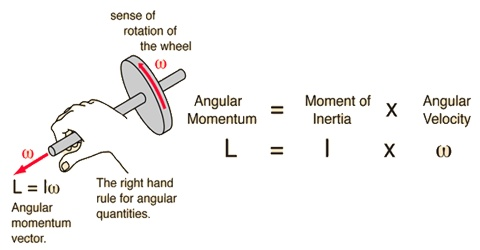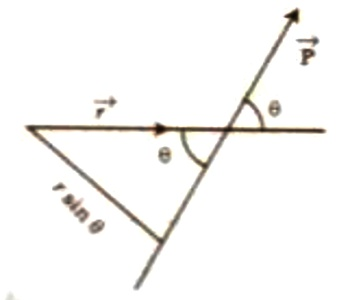# Angular Momentum

Angular Momentum

Definition: Vector product of radius vector of a rotating particle and the linear momentum is called the angular momentum. The angular momentum of a rigid object is defined as the product of the moment of inertia and the angular velocity.

Explanation: Suppose, r = vector radius of a particle with respect to the centre of rotation, and P = linear momentum of the body.

So, according to the definition, angular momentum of the body, L = r x P.

It is a vector quantity.

Magnitude and direction: Magnitude of angular momentum, L = rP Sinθ.

Here θ is the angle between r and P [Figure]. Perpendicular distance of the line of action of the momentum from the centre of rotation is r Sinθ. So, product of the linear momentum of the particle and the perpendicular distance of the line of action from the axis of rotations gives the magnitude of the angular momentum.The direction of L will be along the normal to the plane r and P. This direction [direction of L) is determined by the cross product rule.

Unit and Dimensional Equation: In M.K.S. and S.I. systems unit of angular momentum is kgm2s-1 and dimensional equation,

[L] = [momentum x distance] = [MLT-1L] = [ML2T-1]